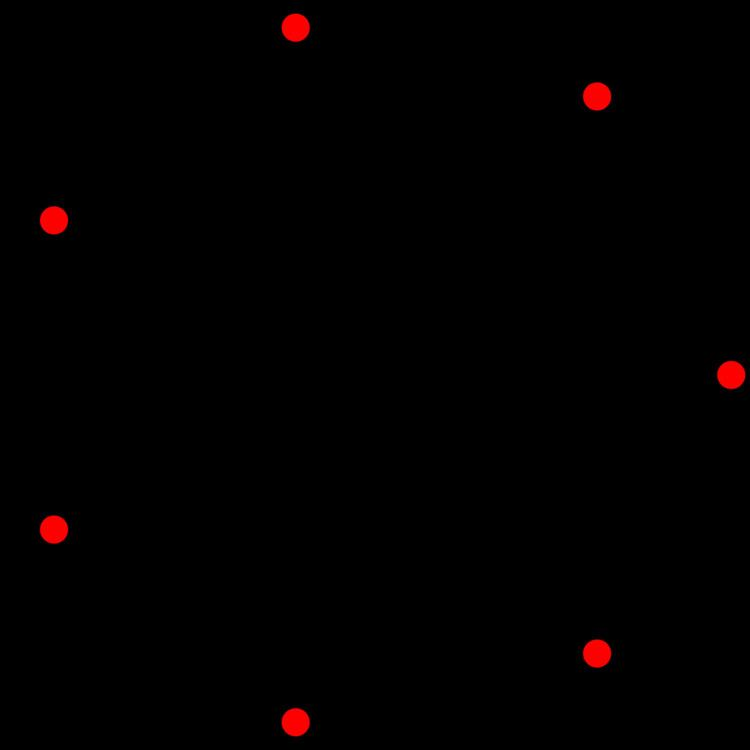# A6 polytope

Updated on
Covid-19In 6-dimensional geometry, there are 35 uniform polytopes with A6 symmetry. There is one self-dual regular form, the 6-simplex with 7 vertices.

Each can be visualized as symmetric orthographic projections in Coxeter planes of the A6 Coxeter group, and other subgroups.

## Graphs

Symmetric orthographic projections of these 35 polytopes can be made in the A6, A5, A4, A3, A2 Coxeter planes. Ak graphs have [k+1] symmetry. For even k and symmetric ringed diagrams, symmetry doubles to [2(k+1)].

These 63 polytopes are each shown in these 5 symmetry planes, with vertices and edges drawn, and vertices colored by the number of overlapping vertices in each projective position.

A6 polytope Wikipedia

Topics